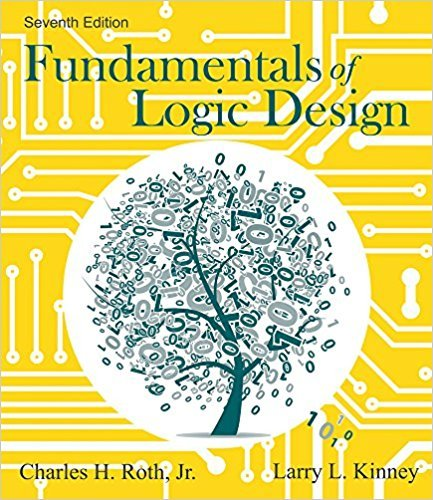×
×

# Solutions for Chapter 16: Sequential Circuit Design## Full solutions for Fundamentals of Logic Design | 7th Edition

ISBN: 9781133628477Solutions for Chapter 16: Sequential Circuit Design

Solutions for Chapter 16
4 5 0 319 Reviews
12
0
##### ISBN: 9781133628477

Chapter 16: Sequential Circuit Design includes 34 full step-by-step solutions. Fundamentals of Logic Design was written by and is associated to the ISBN: 9781133628477. Since 34 problems in chapter 16: Sequential Circuit Design have been answered, more than 31121 students have viewed full step-by-step solutions from this chapter. This textbook survival guide was created for the textbook: Fundamentals of Logic Design, edition: 7. This expansive textbook survival guide covers the following chapters and their solutions.

Key Engineering and Tech Terms and definitions covered in this textbook
×